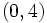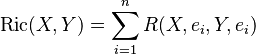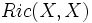# Difference between revisions of "Ricci curvature tensor of Levi-Civita connection"

## Definition

Let$M$ be a differential manifold and$g$ be a Riemannian metric or pseudo-Riemannian metric on$M$. Let$\nabla$ be the Levi-Civita connection associated with$g$. The Ricci curvature tensor of$M$ is defined as the Ricci curvature tensor of the Levi-Civita connection.

Explicitly, if$R$ is the Riemann curvature tensor of the Levi-Civita connection:$\operatorname{Ric}(X,Y) = -Tr(Z \mapsto R(X,Z)Y)$

In the particular case of a Riemannian metric, we can choose an orthonormal basis$e_i$ on each tangent space. For a particular tangent space, if the orthonormal basis is$e_i$, the Ricci curvature tensor evaluated at a pair of vectors$X,Y$ is:$\operatorname{Ric}(X,Y) = \sum_{i=1}^n g(R(X,e_i)Y,e_i)$

Or is the language of$R$ as a$(0,4)$-tensor:$\operatorname{Ric}(X,Y) = \sum_{i=1}^n R(X,e_i,Y,e_i)$

## Related notions

• Ricci curvature in a direction is the Ricci curvature tensor$Ric(X,X)$ where$X$ is a unit vector in that direction

## Facts

### Symmetry

We have:$Ric(X,Y) = Ric(Y,X)$

This follows from the fact that the Riemann curvature tensor is symmetric in the pair of the first two and last two variables. For full proof, refer: Symmetry of Riemann curvature tensor in variable pairs